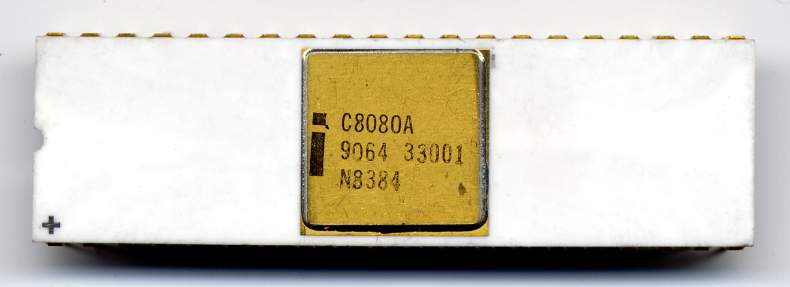## 8080/Z80 Instruction Set

A comparison between Z80 and Intel-8080 assembly instructions.Source: https://es.wikipedia.org/wiki/Intel_8080

DISCLAIMER: This page is a mirror of no longer existing lonestar's site, which is now archived here. This page will be deleted in case of original author's claim. Original work belongs to Frank Durda IV. 8080 Mnemonic Z80 Mnemonic Machine Code Operation MOV A,A LD A,A 7F A <- A MOV A,B LD A,B 78 A <- B MOV A,C LD A,C 79 A <- C MOV A,D LD A,D 7A A <- D MOV A,E LD A,E 7B A <- E MOV A,H LD A,H 7C A <- H MOV A,L LD A,L 7D A <- L MOV A,M LD A,(HL) 7E A <- (HL) LDAX B LD A,(BC) 0A A <- (BC) LDAX D LD A,(DE) 1A A <- (DE) LDA word LD A,(word) 3Aword A <- (word) --- LD A,(IX+index) DD7Eindex A <- (IX+index) --- LD A,(IY+index) FD7Eindex A <- (IY+index) MOV B,A LD B,A 47 B <- A MOV B,B LD B,B 40 B <- B

MOV B,C LD B,C 41 B <- C MOV B,D LD B,D 42 B <- D MOV B,E LD B,E 43 B <- E MOV B,H LD B,H 44 B <- H MOV B,L LD B,L 45 B <- L MOV B,M LD B,(HL) 46 B <- (HL) --- LD B,(IX+index) DD46index B <- (IX+index) --- LD B,(IY+index) FD46index B <- (IY+index) MOV C,A LD C,A 4F C <- A MOV C,B LD C,B 48 C <- B MOV C,C LD C,C 49 C <- C MOV C,D LD C,D 4A C <- D MOV C,E LD C,E 4B C <- E MOV C,H LD C,H 4C C <- H MOV C,L LD C,L 4D C <- L MOV C,M LD C,(HL) 4E C <- (HL) --- LD C,(IX+index) DD4Eindex C <- (IX+index) --- LD C,(IY+index) FD4Eindex C <- (IY+index) MOV D,A LD D,A 57 D <- A MOV D,B LD D,B 50 D <- B MOV D,C LD D,C…